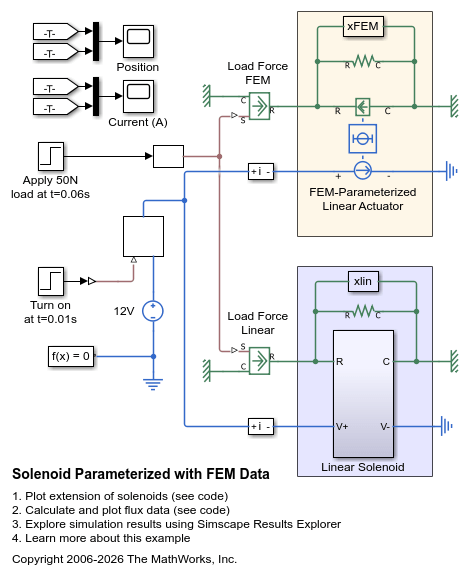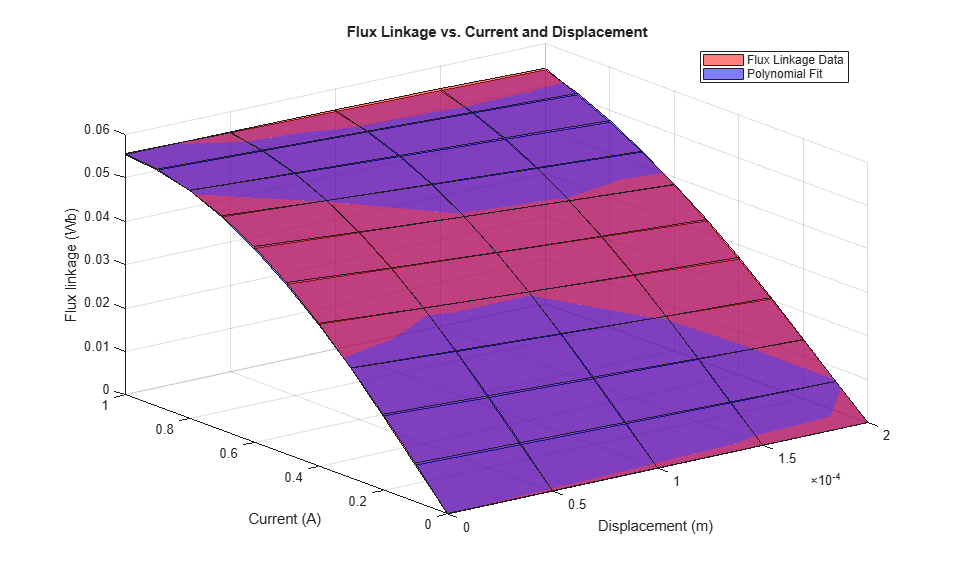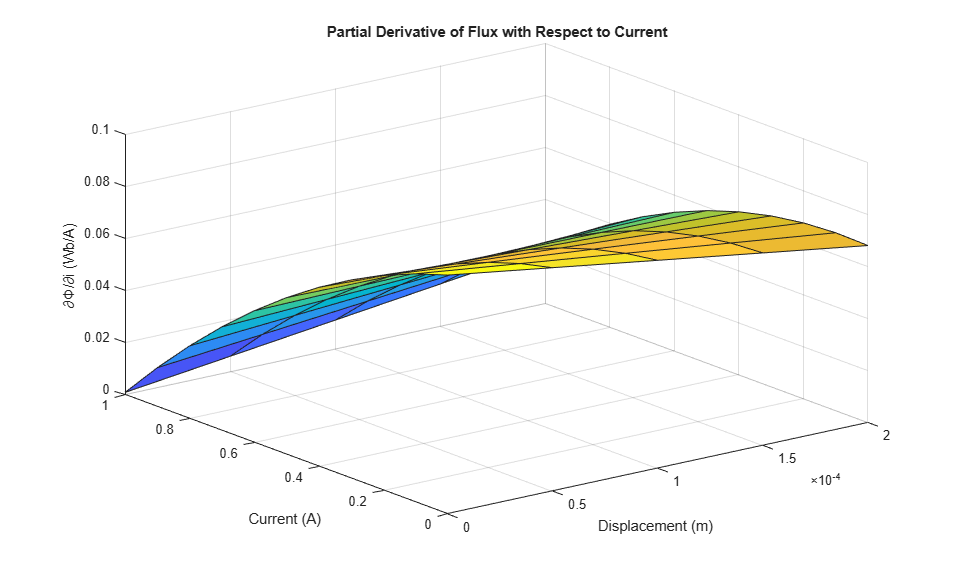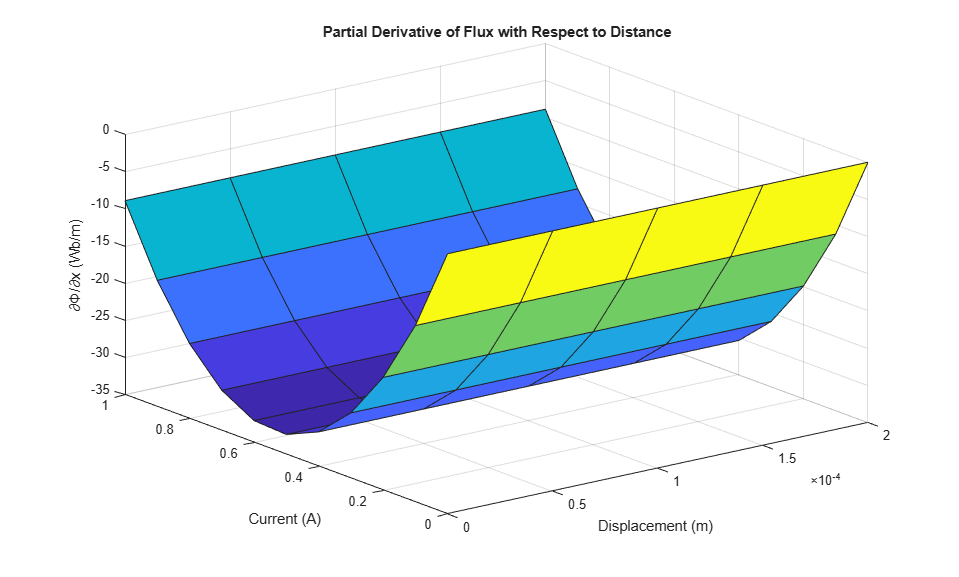# Solenoid Parameterized with FEM Data

This example shows a limited travel solenoid with return spring. When unpowered, the spring holds the plunger at a distance of 0.1mm from the fully energized position. At 0.1 seconds, the solenoid is powered on and the displacement goes to zero. At 0.06s a force higher than the holding force is applied, and the plunger moves to its maximum travel of 0.2mm. The solenoid force and back emf characteristics are defined by the FEM-Parameterized Linear Actuator block. This block takes data in the format typically provided by a finite element magnetic field modeling tool. There are two parameterization options, one which works directly with flux data, and one which uses partial derivatives of flux with respect to current and displacement. The latter option is usually the better choice, it giving more accurate results for a given density of current and position data points. However, it requires more data pre-processing.

A key difference between this approach to modeling a solenoid and the approach used by the Simscape™ solenoid example 'ssc_solenoid' is that it can capture magnetic saturation effects. Conversely the Simscape example assumes a linear relationship between current and magnetic force. This linearity assumption is also made by the Simscape Electrical™ Solenoid component. In this model, the subsystem 'Simplified solenoid with no saturation effects' is parameterized with solenoid inductance data for a current of 0.1A. The inductance is the partial derivative of the flux with respect to current, and is also calculated by the initialization file. The difference in results compared to the linear model of the solenoid illustrates the effects of flux saturation. If you reduce the supply voltage from 12V to 1.4V, then the steady-state current is approximately 0.1A and the results then match once the current has reached this value.

### Model### Simulation Results from Simscape Logging

The plot below shows the difference in behavior between a linear solenoid model and a solenoid model parameterized with data provided from a finite element magnetic field modeling tool.### Simulation Results from Simscape Logging

The plots below show the flux data, including comparisons between methods of calculating the necessary parameters for the FEM-Parameterized Solenoid block.